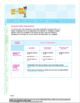Math 6: FULL YEAR BUNDLESubject
Resource Type
Common Core Standards
Product Rating
4.0
3 Ratings
File Type

PDF (Acrobat) Document File

454 KB|+3000 as well as 62 videos, 29 ppts, 51 quizzes and tests, 89 answer keys
Share
Product Description

Ready for the Bell math lessons provide a comprehensive set of print, multimedia resources, assessments and real world learning explorations.

The lessons' instructional design recognizes a new generation of learners, ones that expect multi-media, multi-modal learning opportunities and have the ability to access, research, and master knowledge creation opportunities whether they are at school or at home. At the same time as providing current and multi-modal instructional methods and resources, the lesson provides options for teaching with technology in classrooms that have no tech, some tech or more tech.

Complete Year Bundle Total Inventory:

This complete year bundle includes all lesson packets plus Unit Assessment tests and answer keys for Number Concepts, Algebra (Patterns and Relations), Geometry (Shape and Space), and Statistics. Total counts:

- 542 page teacher guide set

- 1,430 pages of teaching materials and student lessons and workbooks

- 4 contextual animated videos

- 11 instructional notepad tutorial videos

- 14 concept instructional videos

- 14 concept presentation ppts with teaching notes

- 24 lesson quizzes

- 4 unit assessments

LESSON SUMMARIES:

Decimals, Fractions and Rational Numbers

To review each lesson packet, click on the links below:

Lesson 1: Multiplying and Dividing Whole Numbers

Lesson 2: Multiples of Whole Numbers

Lesson 3: Factors of Whole Numbers

Lesson 4: Prime and Composite Numbers

Lesson 5: Multiplying Decimals

Lesson 6: Dividing Decimals

Lesson 7: Multiplication and Division Problem Solving

Lesson 8: Introduction to Integers

Lesson 9: Ordering Integers

Geometry

To review each lesson packet, click on the links below:

Lesson 1: Identifying Prisms

Lesson 2: Constructing Prisms

Ratios and Percents

To review each lesson packet, click on the links below:

Lesson 1: Ratios

Lesson 2: Problem Solving with Ratios

Lesson 3: Percents as a Ratio or Fraction

Lesson 4: Percents as a Decimal

Lesson 5: Problem Solving using Percents

Statistics

To review each lesson packet, click on the links below:

Lesson 1: Describing Outcomes

Lesson 2: Comparing Outcomes

Lesson 3: Possible Outcomes

Lesson 4: Theoretical Probability

Lesson 5: Experimental Probability

Lesson 6: Collecting Data

Lesson 7: Creating a Line Graph

Lesson 8: Analyzing Data

Total Pages
+3000 as well as 62 videos, 29 ppts, 51 quizzes and tests, 89 answer keys
Included
Teaching Duration
1 Year
Report this Resource\$114.00